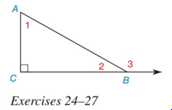Chapter 2.4, Problem 27E### Elementary Geometry for College St...

6th Edition
Daniel C. Alexander + 1 other
ISBN: 9781285195698

#### Solutions

Chapter
Section### Elementary Geometry for College St...

6th Edition
Daniel C. Alexander + 1 other
ISBN: 9781285195698
Textbook Problem
1 views

#In Exercises 25 to 27 , see the figure for exercise 24 . Given: m ∠ 1 = x 2 , m ∠ 2 = x 3 Prove: x

To determine

To find:

The value of x.

Explanation

Given:

m1=x2,

And,

m2=x3

Figure (1)

Calculation:

Sum of acute angles in a right triangle is equal to 90°.

Equate the sum of acute angles in a right triangle to 90°.

m1+m2=90°(1)

Substitute x2 for

### Still sussing out bartleby?

Check out a sample textbook solution.

See a sample solution

#### The Solution to Your Study Problems

Bartleby provides explanations to thousands of textbook problems written by our experts, many with advanced degrees!

Get Started

#### 24. (a) Graph . (b) Graph . (c) Algebraically show why these graphs are identical.

Mathematical Applications for the Management, Life, and Social Sciences

#### Multiply. (1cos)(1+cos)

Trigonometry (MindTap Course List)

#### Show that cosh x1+12x2 for all x.

Single Variable Calculus: Early Transcendentals

#### Sometimes, Always, or Never: If c is a critical number, then f′(c) = 0.

Study Guide for Stewart's Single Variable Calculus: Early Transcendentals, 8th

#### True or False: converges if and only if .

Study Guide for Stewart's Multivariable Calculus, 8th